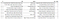# Dataset

`data = pd.read_csv('train.csv')data.head(10)`Dataset — Title is used to predict if an article is fake or not

# Data Preprocessing

`len(data) #20800data.dropna(inplace=True)data.reset_index(inplace=True)len(data) #18285`
`import stringimport nltkstop_words = nltk.corpus.stopwords.words('english')corpus = []`
`def clean_text(data,vocab):    for i in range(len(data)):        txt = data['title'][i]        txt = txt.split()                txt = [word for word in txt if word not in string.punctuation] # Removing punctuations                txt = [word for word in txt if word.isalpha()]#removing all the words having characters other than letters                txt = [word for word in txt if word not in stop_words] #Removing all the stop words                txt = [word.lower() for word in txt] #making all the words lowercase                seq = ' '.join(txt)        split_seq = seq.split()                vocab.update(split_seq)  # Keeping a count                for index in range(len(split_seq)):  # putting all the words in our corpus            corpus.append(split_seq[index])    return corpus`
`from collections import Countervocab = Counter()clean_text(dataTrain,vocab)`
`print(vocab.most_common(100))`
`min_occurence = 20tokens =  [word for word,count in vocab.items() if count>=min_occurence]`
`print(len(tokens)) # 1243print(tokens[:100])`
`def clean_data(txt):    txt = txt.split()    txt = [word for word in txt if word not in string.punctuation]    txt = [word for word in txt if word.isalpha()]    txt = [word for word in txt if word not in stop_words]    txt = [word.lower() for word in txt]    txt = [word for word in txt if word in tokens]    seq = ' '.join(txt)    return seq`
`index = 0labels = []titles = []for index in range(len(data)):    clean_txt = clean_data(data['title'][index]) # cleaning up the title    titles.append(clean_txt.split()) # adding the preprocessed title to our list    labels.append(data['label'][index]) # adding the label for that article`
`print(titles,labels)print(titles,labels)print(titles,labels)print(titles,labels)`
`def create_tokenizer(titles):    tokenizer = Tokenizer()    tokenizer.fit_on_texts(titles)    return tokenizer`
`# Create the tokenizertokenizer = create_tokenizer(titles) X = tokenizer.texts_to_matrix(titles,mode='freq')`
`print(X.shape) # (18285, 1244)y = labels print(y.shape) #(18285,)`
`Xtrain,Ytrain = np.array(Xtrain),np.array(Ytrain)`
`from sklearn.model_selection import train_test_splitX_train,X_test,Y_train,Y_test = train_test_split(Xtrain,Ytrain,test_size=0.25,stratify=Ytrain)`
`print(X_train.shape) #(13713, 1244)print(X_test.shape) # (4572, 1244)print(Y_train.shape) # (4572, 1244)print(Y_test.shape) # (4572, 1244)`

# Model Creation

`from tensorflow.keras.models import Sequentialfrom tensorflow.keras.layers import Densefrom tensorflow.keras.layers import Dropout`
`model = Sequential()   model.add(Dense(50,input_shape=(n_words,),activation='relu'))model.add(Dropout(0.2))    model.add(Dense(100,activation='relu'))model.add(Dropout(0.3))    model.add(Dense(150,activation='relu'))model.add(Dropout(0.4))    model.add(Dense(200,activation='relu'))model.add(Dropout(0.5))    model.add(Dense(1,activation='sigmoid'))    model.compile(loss='binary_crossentropy',optimizer='adam',metrics=['accuracy'])    model.summary()`
`model.fit(X_train,Y_train,epochs=15,verbose=2)`
`model.evaluate(X_test,Y_test)`
`from sklearn.metrics import confusion_matriximport seaborn as snsy_pred = model.predict(X_test)cm = confusion_matrix(Y_test,y_pred)group_names = ['True Neg','False Pos','False Neg','True Pos']group_counts = ['{0:0.0f}'.format(value) for value in                cm.flatten()]group_percentages = ['{0:.2%}'.format(value) for value in                     cm.flatten()/np.sum(cm)]labels = [f"{v1}\n{v2}\n{v3}" for v1, v2, v3 in          zip(group_names,group_counts,group_percentages)]labels = np.asarray(labels).reshape(2,2)sns.heatmap(cm, annot=labels, fmt='', cmap='Blues')`

# Comparing different word scoring models

`def create_tokenizer(X,y,mode):    tk = Tokenizer()    tk.fit_on_texts(X)    X = tk.texts_to_matrix(X,mode=mode)    y = tk.texts_to_matrix(y,mode=mode)    return X,y`
`def evaluate_model(Xtrain,ytrain,Xtest,ytest):    scores = list()    for i in range(10):        model.fit(Xtrain,ytrain,epochs=15,verbose=2)        loss, acc = model.evaluate(Xtest,ytest)        scores.append(acc)    return scores`
`import pandas as pdmodes = ['binary','count','tfidf','freq']results = pd.DataFrame()for mode in modes:    X_train,X_test = create_tokenizer(Xtrain,Xtest,mode)results[mode] = evaluate_model(X_train,ytrain,X_test,ytest)`
`print(results)`
`print(results.describe())`